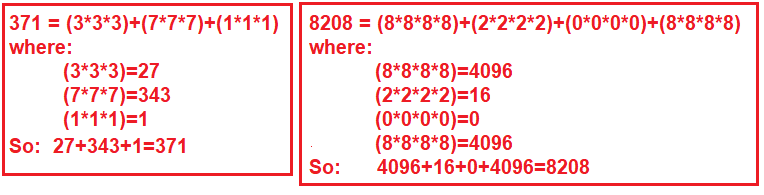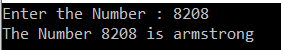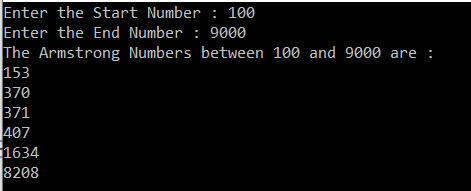# Armstrong Number Program in C#

## Armstrong Number Program in C# with Examples

In this article, I am going to discuss the Armstrong Number Program in C# with Examples. Please read our previous article before proceeding to this article where we discussed the How to Reverse a Number and a String Program in C# with some examples. As part of this article, we are going to discuss the following pointers.

1. What is an Armstrong Number?
2. How to check if a given number is Armstrong or not?
3. Program to find Armstrong numbers between a range of numbers.
##### What is an Armstrong Number?

An Armstrong Number is a number that is equal to the sum of, power of each digit by the total number of digits. For example, the numbers such as 0, 1, 153, 370, 371, and 407, 1634, 8208, 9474 are Armstrong numbers. Let us have a look at the following diagram which shows how the Armstrong number is calculated.##### Let us understand this with an example:

In the following program, first, we are finding the total number of digits in a given number, and storing each digit into an array. And then by using the power method of Math class, we are finding the power of each digit and then calculate the sum of each result. Finally, we are comparing the sum and the input number. If both are equal then it is an Armstrong number else it is not an Armstrong number.

```using System;
namespace LogicalPrograms
{
class Program
{
static void Main(string[] args)
{
int i = 0;
int digitCount = 0;
int[] digitArray = new int;
double sum = 0;

//Step1: Take the input
Console.Write("Enter the Number : ");

//Step3: Store the number in a temporary variable
int temporaryNumber = number;

//Step3: Find the total number of digits in number as well as
//Store each each digit in the digit array
while (number > 0)
{
digitArray[i++] = number % 10;
number = number / 10;
digitCount++;
}

//Step4: Calculate the result
for (i = 0; i < digitCount; i++)
{
sum += Math.Pow(digitArray[i], digitCount);
}

//Step5: Check whether it is prime number or not
if (sum == temporaryNumber)
{
Console.WriteLine(\$"The Number {temporaryNumber} is armstrong");
}
else
{
Console.WriteLine(\$"The Number {temporaryNumber} is not armstrong");
}

}
}
}
```
###### Output:##### Finding Armstrong number between ranges of numbers in C#:

In the following program, we are taking two inputs i.e. start and end numbers from the console and then finding the Armstrong numbers between these two numbers.

```using System;
namespace LogicalPrograms
{
class Program
{
static void Main(string[] args)
{
Console.Write("Enter the Start Number : ");

Console.Write("Enter the End Number : ");

Console.WriteLine(\$"The Armstrong Numbers between {StartNumber} and {EndNumber} are : ");
for (int i = StartNumber; i <= EndNumber; i++)
{
if (IsArmstrongNumber(i))
Console.WriteLine(i);
}

}

static bool IsArmstrongNumber(int number)
{
int sum = 0;
int temporaryNumber = number;
int temp = 0;
int length = number.ToString().Length;

while (number != 0)
{
temp = number % 10;
number = number / 10;
sum += (int)Math.Pow(temp, length);
}

if (sum == temporaryNumber)
{
return true;
}
else
{
return false;
}
}
}
}```
###### Output:In the next article, I am going to discuss the Factorial Number Program in C# with some examples. Here, in this article, I try to explain how to check whether a given number is Armstrong or not in C# with some examples.